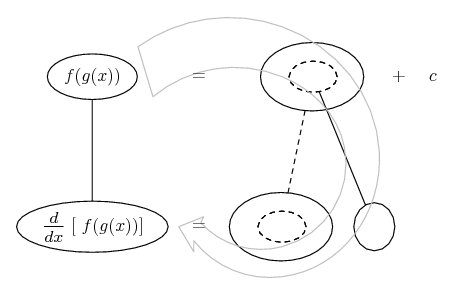# Thread: Rate problem

1. ## Rate problem

A snowball melts in volume @ 1 cm³/min. At what rate is the diameter decreasing when the diameter is 10 cm?
Volume of a sphere: $\displaystyle \frac{4}{3}\pi r^{3}$
Volume of a sphere in terms of diameter: $\displaystyle \frac{\pi d^{3}}{6}$

Rate of volume: $\displaystyle \frac{d}{d(time)}volume = -1 cm^{3}/min.$

Rate of diameter: $\displaystyle \frac{d}{d(time)}10 cm = ?$

I'm not sure what to do at this point

2. ## Re: Rate problem

Let's denote the diameter by D. Then $\displaystyle \frac{dV}{dt}=\frac{dV}{dD}\frac{dD}{dt}$ (d-d-d-t). You know $\displaystyle \frac{dV}{dt}$ and $\displaystyle \frac{dV}{dD}$, so you can find $\displaystyle \frac{dD}{dt}$.

3. ## Re: Rate problemOriginally Posted by emakarovLet's denote the diameter by D. Then $\displaystyle \frac{dV}{dt}=\frac{dV}{dD}\frac{dD}{dt}$ (d-d-d-t).
I don't see how $\displaystyle \frac{dV}{dt}=\frac{dV}{dD}\frac{dD}{dt}$ ?

But I found:
$\displaystyle \frac{dV}{dD} = \frac{\pi}{6} \cdot [D^{3}]' \\ = \frac{\pi}{6} \cdot 3D^{2} \\ = \frac{\pi D^{2}}{2}$

4. ## Re: Rate problem

Just in case a picture helps...... where (key in spoiler) ...

Spoiler:... is the chain rule. Straight continuous lines differentiate downwards (integrate up) with respect to the main variable (in this case t), and the straight dashed line similarly but with respect to the dashed balloon expression (the inner function of the composite which is subject to the chain rule).

The general drift is..._________________________________________

Don't integrate - balloontegrate!

Balloon Calculus; standard integrals, derivatives and methods

Balloon Calculus Drawing with LaTeX and Asymptote!

5. ## Re: Rate problem

Don't you see that we can cancel dD? (Half kidding.) Seriously, this is the chain rule.

6. ## Re: Rate problem

The one thing I am not understanding is where $\displaystyle \frac{dV}{dD}$ came from. How did you know that you need to take the derivative of the volume with respects to the diameter for the problem?

7. ## Re: Rate problem

By seeing that you are trying to differentiate a composite function of t with respect to t. And the chain rule says this will involve differentiating with respect to the whole of the inner function of t. (D is the inner function of t.)

8. ## Re: Rate problem

$\displaystyle \frac{dV}{dt}= \frac{dV}{dD}\frac{dD}{dt}$ is the chain rule. Check your Calculus text.

The problem asks you to find $\displaystyle \frac{dD}{dt}$ and you are told $\displaystyle \frac{dV}{dt}$. "$\displaystyle dV/dD$", which you can easily get from the formula for V in terms of D, is the "missing link" in the chain rule.

9. ## Re: Rate problem

We choose to represent the function V(t) as V(D(t)). The chain rule says that $\displaystyle \frac{dV}{dt}=\frac{dV}{dD}\frac{dD}{dt}$.

Properly speaking, where something came from is not a mathematical question. The solution to this question is a sequence of statements, each of which is either true or false (hopefully, true). The only proper question is why a certain statement is true.

Edit: Yes, it's legitimate to ask metamathematical questions, such as what reasoning one used to come up with a certain solution. Hopefully, post #8 answered this question.

10. ## Re: Rate problem

It makes more sense to use the Chain rule now that I see there is a function V(D(t)). I did not know this was the function that I was supposed to use to solve the problem. The issue I am having is how to formulate the problem so I can solve it. I did not know to express the volume as the diameter when the time changes the diameter...I am still a bit confused as to how I can get this, but I think I will skip this one for now and come back to it later because I have other problems I need to finish as well as other classes.

11. ## Re: Rate problem

Oh, I see now. Taking the derivative of the Volume with respect to time:

$\displaystyle \frac{dV}{dt} = [\frac{\pi d^{3}}{6}]'$

However, taking the derivative of the diameter results in using the Chain rule which I get.

I just didn't know how to come up with the formula: $\displaystyle \frac{dV}{dt} = [\frac{\pi d^{3}}{6}]'$ to solve this. All I needed was to know how to arrive at $\displaystyle \frac{dV}{dt} = [\frac{\pi d^{3}}{6}]'$ which I didn't know how to in the first place.

12. ## Re: Rate problemOriginally Posted by daigoIt makes more sense to use the Chain rule now that I see there is a function V(D(t)). I did not know this was the function that I was supposed to use to solve the problem.
There is never a function that your are supposed to use to solve a problem- look at all functions and formulas that have anything to do with the problem.
In this case you are told that "a snowball melts in volume @ 1 cc/min" so the rate of change of V with respect to t is surely relevant.

The issue I am having is how to formulate the problem so I can solve it. I did not know to express the volume as the diameter when the time changes the diameter...I am still a bit confused as to how I can get this, but I think I will skip this one for now and come back to it later because I have other problems I need to finish as well as other classes.

13. ## Re: Rate problemOriginally Posted by daigoAll I needed was to know how to arrive at $\displaystyle \frac{dV}{dt} = [\frac{\pi d^{3}}{6}]'$ which I didn't know how to in the first place.
Think of it as balancing.

V = f(d)

would imply

3*V = 3*f(d)

and

2 + V = 2 + f(d)

etc... ?

So wouldn't it imply...

$\displaystyle \frac{d}{dt} V = \frac{d}{dt} f(d)$

... ?

#### Search Tags

problem, rate